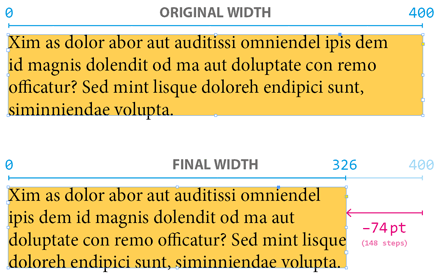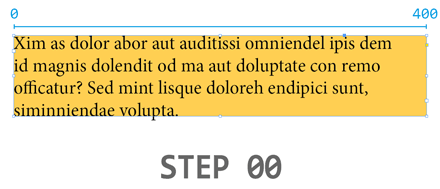Here is the deal: you need to fit a `TextFrame` to its content without changing its height. In other words, taking into account the original state of the object, you want to find the minimal width that still prevents the frame from overflowing.

The naive approach is to reduce the length bit by bit until you reach the limit. But the reduction step must be small enough to meet the required precision, say 0.5pt, which leads to a huge number of steps when the solution is far from the initial state. Here is the picture:In the above example 148 steps are needed to reach the optimal width. Note that at least two InDesign DOM commands are sent at each step: the first for changing the frame width (i.e. its `geometricBounds`), the second for checking the `overflows` flag.

Now consider the binary search algorithm. It begins by testing the middle value, that is the half width. If the value is too small (i.e. the text frame overflows), then the process continues on the upper half, otherwise it continues on the lower half. At each iteration the search interval is divided by two, which somehow eliminates half of the possible values. In mathematical terms, W being the initial width and P being the required precision (expressed in the same unit) there are `N = W/P` possible results. Then, on average, the step-by-step method will reach the solution in `N/2` iterations, while the binary search will reach it in `log2(N)+1` iterations.

In my example `W=400`, `P=0.5` so `N=800`. Hence the naive method involves on average 400 iterations (actually, 148) while the divide-and-conquer process only involves `|log2(800)+1| = 11` iterations (actually, 10), as shown in the animation below:Note. — A possible implementation of this fitHorizontal routine is provided in the entry “Fit Text Frame to Content (supporting multicolumn)” of the ISFR series.

## Implementation Notes

Binary search algorithms are easy to implement. They only require you backup the current bounds of the search interval. At each iteration the middle term is calculated and takes the place of either the lower or the upper bound depending on some condition. I often use a `[min,max]` array `a` to represent the in-progress interval. The middle value `mid` is therefore `(a+a)/2`. Now suppose that a `checkState()` function returns either `0` or `1`, where `1` means that `mid` is too high at the current stage—for example: `+myFrame.overflows`. One can then use `a[checkState()]=mid` to update the array and divide the interval accordingly.

Here is a skeleton:

```var findOptimalValue = function(...)
{
// Settings
// ---
const MIN = ...,
MAX = ...,
PRECISION = ...;

// Initialization
// ---
var a = [MIN,MAX],
mid;

// Dichotomic search
// ---
while( a-a > PRECISION )
{
mid = (a+a)/2;
a[checkState()] = mid;
}

// Conclusion
// ---
// Depending on your requirements, return mid, a, or a
};
```

A basic example is provided in this maximizePointSize function. The goal was to find the maximum point size for the text contained in a given frame. As you will see, the dichotomic algorithm has many applications in adjusting stuff with respect to metric constraints (dimensions, point size, tracking, scaling, etc.)

See also the entry “Fit Horizontal Text Scale to Frame” in ISFR #8. It provides a very abstract snippet for various optimal adjustment problems.

Here is a more sophisticated use of the divide-and-conquer process. The following function returns a fractional representation of a decimal number within `[0..1]`. It uses the Stern–Brocot binary tree to find quickly the optimal result, as suggested in this discussion.

```var fractionalForm = function(/*0..1*/x,  xMin,xMax,n,d,mn,md)
{
const EPSILON = .00001;

if( (xMin=x-EPSILON) < 0 ) return '0';
if( (xMax=x+EPSILON) > 1 ) return '1';

n = [0,1];
d = [1,1];

while( 1 )
{
mn = n + n;
md = d + d;

if( md*xMax < mn )
{
n = mn;
d = md;
continue;
}

if( md*xMin > mn )
{
n = mn;
d = md;
continue;
}

return mn + '/' + md;
}
};